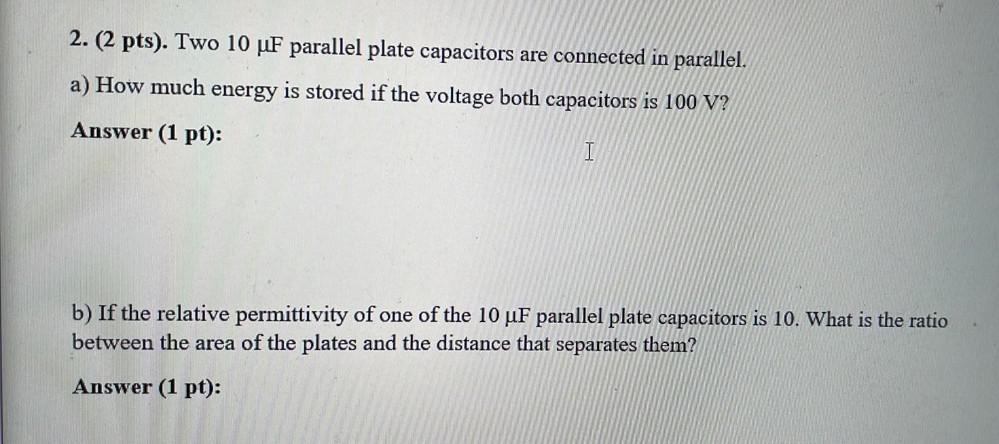Question:

# Two 10 μF parallel plate capacitors are connected in parallel. (A) How much energy is stored if the voltage both capacitors is 100 V? (B) If the relative permittivity of one of the 10 μF parallel plaTwo 10 μF parallel plate capacitors are connected in parallel. (A) How much energy is stored if the voltage both capacitors is 100 V? (B) If the relative permittivity of one of the 10 μF parallel plate capacitors is 10. What is the ratio between the area of the plates and the distance that separates them?